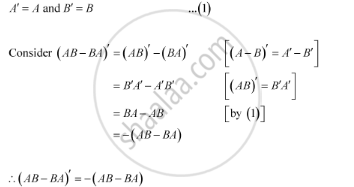Share

# If A, B Are Symmetric Matrices of Same Order, Then Ab − Ba Is a - CBSE (Science) Class 12 - Mathematics

#### Question

If AB are symmetric matrices of same order, then AB − BA is a

A. Skew symmetric matrix B. Symmetric matrix

C. Zero matrix D. Identity matrix

#### Solution

A and B are symmetric matrices, therefore, we have:Thus, (AB − BA) is a skew-symmetric matrix.

Is there an error in this question or solution?

#### APPEARS IN

Solution If A, B Are Symmetric Matrices of Same Order, Then Ab − Ba Is a Concept: Order of a Matrix.
S